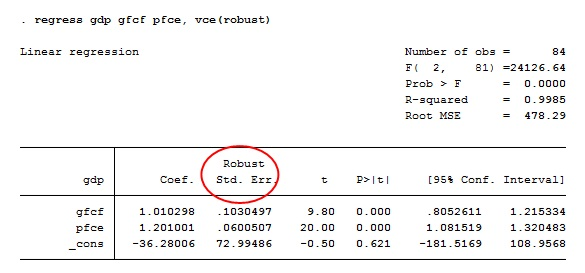# How to perform Heteroscedasticity test in STATA for time series data?

The previous articles showed how to perform normality tests in time series data. This article focuses on another important diagnostic test, i.e. heteroscedasticity test in STATA. Heteroskedastic means “differing variance” which comes from the Greek word “hetero” (‘different’) and “skedasis” (‘dispersion’). It refers to the variance of the error terms in a regression model in an independent variable.

If heteroscedasticity is present in the data, the variance differs across the values of the explanatory variables and violates the assumption. This will make the OLS estimator unreliable due to bias. It is therefore imperative to test for heteroscedasticity and apply corrective measures if it is present. Various tests help detect heteroscedasticities such as Breusch Pagan test and White test.

Heteroscedasticity tests use the standard errors obtained from the regression results. Therefore, the first step is to run the regression with the same three variables considered in the previous article for the same period of 1997-98 to 2017-18.

## Regression results

The previous article explained the procedure to run the regression with three variables in STATA. The regression result is as follows.Figure 1: Regression results for 3 variables

Now proceed to the heteroscedasticity test in STATA using two approaches.

## Breusch-Pagan test for heteroscedasticity

Breusch-Pagan test helps to check the null hypothesis versus the alternative hypothesis. A null hypothesis is that where the error variances are all equal (homoscedasticity), whereas the alternative hypothesis states that the error variances are a multiplicative function of one or more variables (heteroscedasticity).To perform Breusch Pagan test use this STATA command:

`estat hettest`

The below results will appear.Figure 2: Results from Breusch-Pagan test

The figure above shows that the probability value of the chi-square statistic is less than 0.05. Therefore the null hypothesis of constant variance can be rejected at 5% level of significance. It implies the presence of heteroscedasticity in the residuals.

## White test for heteroscedasticity

To check heteroscedasticity using White test, use the following command in STATA:

`estat imtest, white`

The below results will appear.Figure 3: Results from the White test using STATA

Similar to the results of the Breusch-Pagan test, here too prob > chi2 = 0.000. The null hypothesis of constant variance can be rejected at 5% level of significance. The implication of the above finding is that there is heteroscedasticity in the residuals.

## Graphical depiction of results from heteroscedasticity test in STATA

Present heteroscedasticity graphically using the following procedure (figure below):

• Go to ‘Graphics’
• Selecting ‘Regression diagnostic plots’
• Choose ‘Residuals-versus-fitted’.Figure 4: Selection of residuals versus fitted

The rvfplot box will appear (figure below). Click on ‘Reference lines’. Click on ‘OK’.Figure 5: Selecting reference lines for heteroscedasticity test in STATA

The ‘Reference lines (y axis)’ window will appear (figure below). Enter ‘0’ in the box for ‘Add lines to the graph at specified y axis values’. Then click on ‘Accept’.Figure 6: Dialogue box after selecting reference lines

The following graph will appear.Figure 7: Residuals versus fitted plot for heteroscedasticity test in STATA

The above graph shows that residuals are somewhat larger near the mean of the distribution than at the extremes. Also, there is a systematic pattern of fitted values.

## Presence of heteroscedasticity

Thus heteroscedasticity is present. This can be due to measurement error, model misspecifications or subpopulation differences. Consequences of the heteroscedasticity are that the OLS estimates are no longer BLUE (Best Linear Unbiased Estimator). Standard errors will be unreliable, which will further cause bias in test results and confidence intervals.

Therefore correct heteroscedasticity either by changing the functional form or by using a robust command in the regression.

## Correction for heteroscedasticity

In order to get the robust standards errors, add the ‘vce (robust)’ command after the regression:

regress gdp gfcf pfce, vce(robust)
This will output the following result (figure below).Figure 8: Regression results after correction in the heteroscedasticity test in STATAThus the problem of heteroscedasticity is not present anymore. This gives robust standards errors, which are different from standard errors in figure 1. Here robust standard error for the variable gfcf is 0.1030497, which is different from 0.076651 given by figure 1. Similar is the case with the variable pfcf.

The next article explains the test for autocorrelation. Presence of autocorrelation or serial correlation is a violation of another important ordinary least squares (OLS) assumption that errors in the regression model are uncorrelated with each other at all the points in time.

### Rashmi Sajwan

Research Analyst at Project Guru
Rashmi has completed her bachelors in Economic (hons.) from Delhi University and Masters in economics from Guru Gobind Singh Indrapastha University. She has good understanding of statistical softwares like STATA, SPSS and E-views. She worked as a Research Intern at CIMMYT international maize and wheat improvement centre. She has an analytical mind and can spend her whole day on data analysis. Being a poetry lover, she likes to write and read poems. In her spare time, she loves to do Dance.

#### Latest posts by Rashmi Sajwan (see all)

Discussions

1.Anju

Hi,
My name is Anju. I am trying to do a VAR model. My residual plot shows that my model has hetroskedasticity. How can I solve this problem in VAR model . I am using Stata. My variables have a lot of negative values , so they cannot be changed into log form. In this situation, how can I solve the problem of hetroskedasticity? I hope you can help me !

2.Dr Lee

No.

You CAN’T. (Dr Lee)

3.Lu, Yin-Chieh

In Figure 7: Residuals versus fitted plot for heteroscedasticity test in STATT
need I all the variable heteroscedasticity

4.sasika

hello i need interpretation for robust analysis which means how we can identify that heteroscedasticity was solvedWe are looking for candidates who have completed their master's degree or Ph.D. Click here to know more about our vacancies.